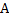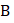# A uniform rod of massand lengthis placed over a smooth horizontal surface along the-axis and is at rest as shown in Figure. An impulsive forceis applied for a small timealong-direction at point. The-coordinate of endof the rod when the rod become parallel to-axis for the first time is [initially, the coordinate of centre of mass of the rod isa)b)c)d)s

## Question ID - 100226 :- A uniform rod of massand lengthis placed over a smooth horizontal surface along the-axis and is at rest as shown in Figure. An impulsive forceis applied for a small timealong-direction at point. The-coordinate of endof the rod when the rod become parallel to-axis for the first time is [initially, the coordinate of centre of mass of the rod isa)b)c)d)s

3537

 (d) As torque =change in angular momentum(linear momentum)    …(i) and(angular momentum)   …(ii) Dividing Eqs. (i) and (ii), we getUsingDisplacement of CM isandDividing, we getCoordination ofwill beNext Question :

Two men ‘’ and ‘’ are standing on a plank. ‘’ is at the middle of the plank andis at the left end of the plank. Lower surface of the plank is smooth. System is initially at rest and masses are as shown in fig. ‘’  and ‘’ start moving such that the position ofremains fixed with respect to ground, then ‘’ meetsThen the point wheremeetsis located ata) The middle of the plank b) 30 cm from the left end of the plank c) The right end of the plank d) None of these